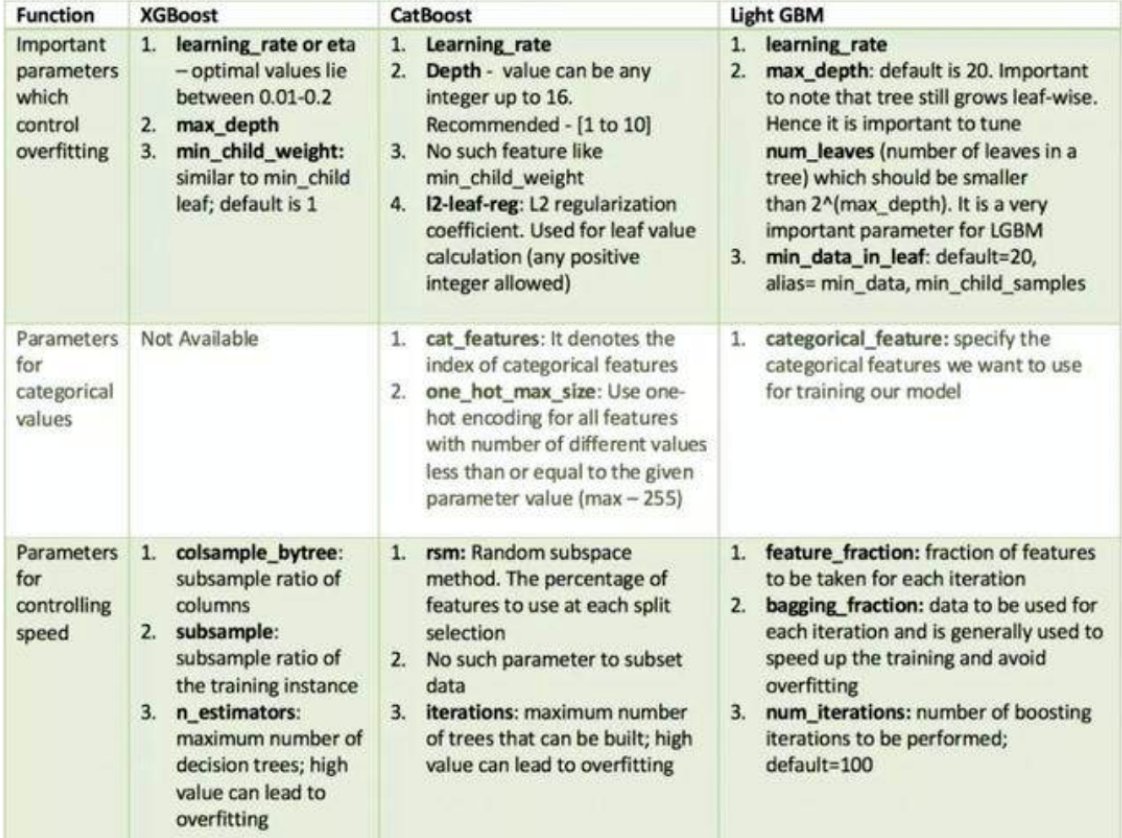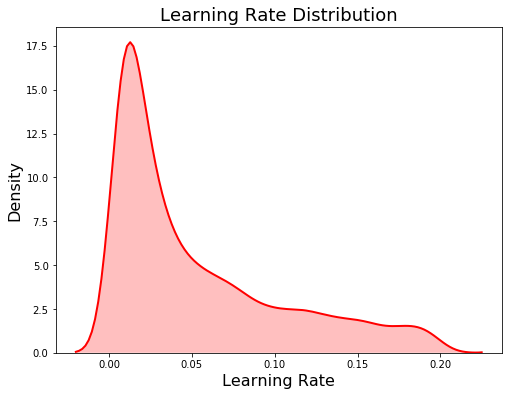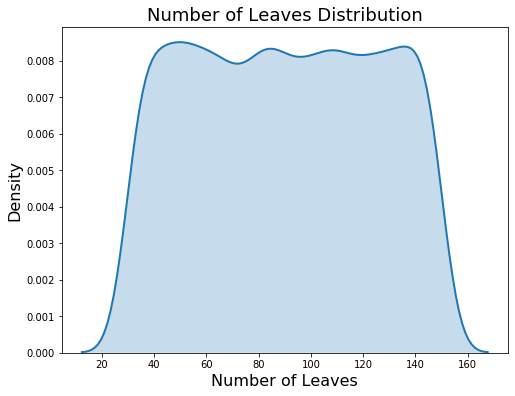• GBDT原始论文+XGB原始论文+陈天奇 ppt
• LS产电XGB系列PLC产品样本zip,LS产电XGB系列PLC产品样本
• 使用XGB算法对旅客航空信息进行特征筛选，分析，分类处理。
• 1 问题描述 我想用XGBoost来建立一个模型，通过特征...xgb = XGBRegressor() xgb.fit(X, Y) print(xgb.feature_importances_) plt.figure(figsize=(20, 10)) plot_importance(xgb) plt.show() 输出如下： 这里输出
• xgb2sql CRAN 发布 构建状态 包装小插曲 描述 此包通过将训练好的模型对象转换为 SQL 查询，可以对 R 中内置的 XGBoost 模型进行数据库内评分。 安装 最新的 CRAN 版本： install.packages( " xgb2sql " ) 开发版： ...
• 经历数据竞赛后，大佬们的总结源码，学习思考后整理除一些源码，语言用python编写
• 陈天奇xgb论文。Tree boosting is a highly eective and widely used machine learning method. In this paper, we describe a scalable endto- end tree boosting system called XGBoost, which is used widely by ...
• XGB位置控制模块用户手册zip,XGB位置控制模块用户手册
• 改进的 XGBoost 类似 scikit-learn 的界面 关于 scikit-learn 是 Python 的机器学习库。 XGBoost 是一个新的有用的梯度提升库，它提供了一个定制的 Python 界面以及一个简化的 scikit-learn-like 界面。...Python
• 这是我们团队用来与 xgb 模型结合的最佳神经网络模型。 单个 NN 模型可以给出大约 0.44（logloss）的排行榜，而 10NN 的简单线性组合将给出大约 0.43。 此模型使用的功能包括以下内容： 辍学层（p = 0.15、0.25、...Python
• LS产电XGB-U型样本zip,LS产电XGB-U型样本
• ## xgb调参--简洁版

千次阅读 2020-04-28 21:50:36
树越深也会消耗更多内存且会使得训练时间变长（由于xgb会一直分裂到max_depth指定的值，再回过头来剪枝） 2、eta[默认0.3] 学习率，常用0.01~0.5之间 太大准确率不高、难以收敛(梯度值可能在最优解附近晃荡，不...
目录
一、常调参数
1、max_depth[默认6]
2、eta[默认0.3]
3、lambda[默认1]
4、alpha[默认0]
5、min_child_weight[默认1]
6、subsample[默认1]
7、colsample_bytree[默认1]
8、gamma[默认0]
9、scale_pos_weight[默认1]
10、tree_method [默认auto]
11、num_boost_round—迭代次数
12、early_stopping_rounds
二、调参方向/目的
1、过拟合
2、优化性能
3、正负样本不均衡
三、调参方法
1、sklearn.model_selection.GridSearchCV——（网格搜索）
2、sklearn.model_selection.RandomizedSearchCV——（随机搜索）
3、hyperopt——（贝叶斯优化）
四、xgb各种参数含义

一、常调参数
1、max_depth[默认6]
树分裂最大深度，常用3~10之间
树越深越容易过拟合（越深模型会学到越具体越局部的样本）
树越深也会消耗更多内存且会使得训练时间变长（由于xgb会一直分裂到max_depth指定的值，再回过头来剪枝）
2、eta[默认0.3]
学习率，常用0.01~0.5之间
太大准确率不高、难以收敛(梯度值可能在最优解附近晃荡，不收敛)
太小运行速度慢
经验：learning_rate * num_round >= 1 and  learning_rate * num_round <= 10
3、lambda[默认1]
权重的L1正则化项
4、alpha[默认0]
权重的L2正则化项
5、min_child_weight[默认1]
最小叶子节点样本权重和(叶子节点中的样本二阶导求和)
值较大时，可减少过拟合
值过高，会导致欠拟合
6、subsample[默认1]
每棵树随机采样的比例，常用0.5~1之间
减少此参数值，算法会更加保守写，以避免一定程度过拟合，但值太小容易欠拟合
7、colsample_bytree[默认1]
控制每棵随机采样的列数的比例(每一列一个特征)，常用0.5~1之间
8、gamma[默认0]
节点分裂所需的最先损失下降
值越大，算法越保守
9、scale_pos_weight[默认1]
设置正样本权重值，以均衡正负样本权重
A typical value to consider: sum(negative instances) / sum(positive instances)
示例见https://github.com/dmlc/xgboost/blob/master/demo/kaggle-higgs/higgs-numpy.py
10、tree_method [默认auto]
树构造算法，可选auto, exact, approx, hist, gpu_hist,通常用于调整训练速度
auto：启发式选择最快的算法，表现为小数据选exact greedy，大数据选approx，建议大数据尝试使用hist或者gpu_hist,以获得更高的性能，gpu_hist支持外部内存
exact：精确贪婪算法，枚举所有候选项
approx：近似贪婪算法，using quantile sketch and gradient histogram(分位数简化图和梯度直方图)
hist：快速直方图优化approx
gpu_hist：hist算法的GPU实现
11、num_boost_round—迭代次数
12、early_stopping_rounds
迭代过程中，在n轮内是否有进步，没有就停止训练
触发这个参数（也就是确实提前停止了）的时候返回的变量会带有3个属性：best_score, best_iteration, best_ntree_limit ，这里best_ntree_limit 就是最好的模型的树的个数
但是在文档和源码中，有这么一句话 The method returns the model from the last iteration (not the best one). 就是说如果触发了这个参数的话，那么结果返回的并不是最好的模型，而是最后一轮的模型，那这不坑爹呢？！
但是后续再深入测试的时候发现，用各种指标去验证（比如rmse）的时候，结果却和最好的模型是一样的，并不是和最后一轮的模型一样，再深入的研究之后在源码中发现了这么一段代码，XGBoost在调用predict的时候tree_limit参数如果没指定默认用的就是best_ntree_limit，也就是在预测时候，用的还是最好的模型
二、调参方向/目的
1、过拟合
直接调整控制模型复杂度参数
max_depth --> 调低min_child_weight --> 调高gamma --> 调高增加随机性，使得训练对噪声具有鲁棒性
subsample  --> 调低colsample_bytree  --> 调低eta and num_round  --> 调低eta,调高num_round
2、优化性能
tree_method, 设置为 hist 或者 gpu_hist来加快计算速度
3、正负样本不均衡
如果想优化整体效果(AUC)
设置正样本权重系数scale_pos_weight使用AUC作为评估标准如果想提升准确率率(预测正确的概率)

设置max_delta_step为1-10之间，有助于收敛
三、调参方法
常用集成学习比较好总要需要调参的参数：1、sklearn.model_selection.GridSearchCV——（网格搜索）
class sklearn.model_selection.GridSearchCV(estimator, param_grid, scoring=None, fit_params=None, n_jobs=1, iid=True, refit=True, cv=None, verbose=0, pre_dispatch=‘2*n_jobs’, error_score=’raise’, return_train_score=’warn’)
import numpy as np
import pandas as pd
import xgboost as xgb
from sklearn.model_selection import GridSearchCV

#导入训练数据
traindata = traindata.set_index('instance_id')
print(traindata.shape,trainlabel.shape)

#分类器使用 xgboost
clf1 = xgb.XGBClassifier()

#设定网格搜索的xgboost参数搜索范围，值搜索XGBoost的主要6个参数
param_dist = {
'n_estimators':range(80,200,4),
'max_depth':range(2,15,1),
'learning_rate':np.linspace(0.01,2,20),
'subsample':np.linspace(0.7,0.9,20),
'colsample_bytree':np.linspace(0.5,0.98,10),
'min_child_weight':range(1,9,1)
}

#GridSearchCV参数说明，clf1设置训练的学习器
#param_dist字典类型，放入参数搜索范围
#scoring = 'neg_log_loss'，精度评价方式设定为“neg_log_loss“
#n_iter=300，训练300次，数值越大，获得的参数精度越大，但是搜索时间越长
#n_jobs = -1，使用所有的CPU进行训练，默认为1，使用1个CPU
grid = GridSearchCV(clf1,param_dist,cv = 3,scoring = 'neg_log_loss',n_iter=300,n_jobs = -1)

#在训练集上训练
grid.fit(traindata.values,np.ravel(trainlabel.values))
#返回最优的训练器
best_estimator = grid.best_estimator_
print(best_estimator)
#输出最优训练器的精度

#自定义损失函数logloss
#===============================我是华丽丽的分割线===============================
import numpy as np
from sklearn.metrics import make_scorer
import scipy as sp

def logloss(act, pred):
epsilon = 1e-15
pred = sp.maximum(epsilon, pred)
pred = sp.minimum(1-epsilon, pred)
ll = sum(act*sp.log(pred) + sp.subtract(1, act)*sp.log(sp.subtract(1, pred)))
ll = ll * -1.0/len(act)
return ll

#这里的greater_is_better参数决定了自定义的评价指标是越大越好还是越小越好
loss = make_scorer(logloss, greater_is_better=False)
score = make_scorer(logloss, greater_is_better=True)
2、sklearn.model_selection.RandomizedSearchCV——（随机搜索）
sklearn.model_selection.RandomizedSearchCV( estimator, param_distributions, n_iter=10, scoring=None, n_jobs=None, iid='deprecated', refit=True, cv=None, verbose=0, pre_dispatch='2*n_jobs', random_state=None, error_score=nan, return_train_score=False, )
RandomizedSearchCV的使用方法其实是和GridSearchCV一致的，但它以随机在参数空间中采样的方式代替了GridSearchCV对于参数的网格搜索，在对于有连续变量的参数时，RandomizedSearchCV会将其当作一个分布进行采样这是网格搜索做不到的，它的搜索能力取决于设定的n_iter参数（数值越大，获得的参数精度越大，但是搜索时间越长
import numpy as np
import pandas as pd
import xgboost as xgb
from sklearn.grid_search import RandomizedSearchCV

#导入训练数据
traindata = traindata.set_index('instance_id')
print(traindata.shape,trainlabel.shape)

#分类器使用 xgboost
clf1 = xgb.XGBClassifier()

#设定搜索的xgboost参数搜索范围，值搜索XGBoost的主要6个参数
param_dist = {
'n_estimators':range(80,200,4),
'max_depth':range(2,15,1),
'learning_rate':np.linspace(0.01,2,20),
'subsample':np.linspace(0.7,0.9,20),
'colsample_bytree':np.linspace(0.5,0.98,10),
'min_child_weight':range(1,9,1)
}

#RandomizedSearchCV参数说明，clf1设置训练的学习器
#param_dist字典类型，放入参数搜索范围
#scoring = 'neg_log_loss'，精度评价方式设定为“neg_log_loss“
#n_iter=300，训练300次，数值越大，获得的参数精度越大，但是搜索时间越长
#n_jobs = -1，使用所有的CPU进行训练，默认为1，使用1个CPU
grid = RandomizedSearchCV(clf1,param_dist,cv = 3,scoring = 'neg_log_loss',n_iter=300,n_jobs = -1)

#在训练集上训练
grid.fit(traindata.values,np.ravel(trainlabel.values))
#返回最优的训练器
best_estimator = grid.best_estimator_
print(best_estimator)
#输出最优训练器的精度
print(grid.best_score_)
3、hyperopt——（贝叶斯优化）
网格搜索速度慢，但在搜索整个搜索空间方面效果很好，而随机搜索很快，但可能会错过搜索空间中的重要点。
hyperopt：是python中的一个用于"分布式异步算法组态/超参数优化"的类库。使用它几乎可以摆脱繁杂的超参数优化过程，自动获取最佳的超参数。广泛意义上，可以将带有超参数的模型看作是一个必然的非凸函数，因此hyperopt几乎可以稳定的获取比手工更加合理的调参结果。尤其对于调参比较复杂的模型而言，其更是能以远快于人工调参的速度同样获得远远超过人工调参的最终性能。
def hyperopt_eval_func(params, X, y):
'''利用params里定义的模型和超参数，对X进行fit，并返回cv socre。
Args:
@params: 模型和超参数
@X:输入参数
@y:真值
Return:
@score: 交叉验证的损失值
'''

int_feat = ['n_estimators', 'max_depth', 'min_child_weight']
for p in int_feat:
params[p] = int(params[p])

clf = XGBClassifier(**params)

#用cv结果来作为评价函数
from sklearn.model_selection import KFold
shuffle = KFold(n_splits=5, shuffle=True)
score = -1 * cross_val_score(clf, X, y, scoring='f1', cv=shuffle).mean()

return score

def hyperopt_binary_model(params):
'''hyperopt评价函数，在hyperopt_eval_func外面包围了一层，增加一些信息输出
Args:
@params:用hyperopt调参优化得到的超参数
Return:
@loss_status: loss and status

'''
global best_loss, count, binary_X, binary_y
count += 1

clf_type = params['type']
del params['type']
loss = hyperopt_eval_func(params, binary_X, binary_y)
print(count, loss)
if loss < best_loss:
ss = 'count:%d new best loss: %4.3f , using %s'%(count, loss, clf_type)
print(ss)
best_loss = loss

loss_status = {'loss': loss, 'status': STATUS_OK}
return loss_status

def get_best_model(best):
'''根据hyperopt搜索的参数，返回对应最优score的模型
Args:
@best:最优超参数
Return:
@clf: xgb model
'''
int_feat = ['n_estimators', 'max_depth', 'min_child_weight']
for p in int_feat:
best[p] = int(best[p])

#fix the random state
best['seed'] = 2018
clf = XGBClassifier(**best)

return clf

def get_best_model(X_train, y_train, predictors, max_evals_num=10):
'''利用hyperopt得到最优的xgb model
Args:
@X_train: 训练样本X 数据
@y_train: 训练样本y target
@predictors: 用于预测的特征
@max_evals_num: hyperopt调参时的次数，次数越多，模型越优，但是也越耗费时间
Return:
@clf: 最优model
'''
space = {
'type': 'xgb',
'n_estimators': hp.quniform('n_estimators', 50,400,50), ##50~400，每间隔50
'max_depth': hp.quniform('max_depth', 2, 8, 1),
##'learning_rate': hp.loguniform('learning_rate', np.log(0.005), np.log(0.2))
'learning_rate': hp.uniform('learning_rate', 0.01, 0.1),
'min_child_weight': hp.quniform('min_child_weight', 2, 8, 1),
'gamma': hp.uniform('gamma', 0, 0.2),
'subsample': hp.uniform('subsample', 0.7, 1.0),
'colsample_bytree': hp.uniform('colsample_bytree', 0.7, 1.0)
}

#hyperopt train
global count, best_loss, binary_X, binary_y
count = 0
best_loss = 1000000
binary_X = X_train
binary_y = y_train
trials = Trials()
best = fmin(hyperopt_binary_model, space, algo=tpe.suggest, max_evals=max_evals_num, trials=trials)
print( 'best param:{}'.format(best))
print('best trans cv mse on train:{}'.format(best_loss))

clf = get_best_model(best)

return clf
定义参数空间可选择函数：
hp.pchoice(label,p_options)以一定的概率返回一个p_options的一个选项。这个选项使得函数在搜索过程中对每个选项的可能性不均匀。hp.uniform(label,low,high)参数在low和high之间均匀分布。hp.quniform(label,low,high,q),参数的取值round(uniform(low,high)/q)*q，适用于那些离散的取值。hp.loguniform(label,low,high) 返回根据 exp（uniform（low，high）） 绘制的值，以便返回值的对数是均匀分布的。 优化时，该变量被限制在[exp（low），exp（high）]区间内。hp.randint(label,upper) 返回一个在[0,upper)前闭后开的区间内的随机整数。hp.normal(label, mu, sigma) where mu and sigma are the mean and standard deviation σ , respectively. 正态分布，返回值范围没法限制。hp.qnormal(label, mu, sigma, q)hp.lognormal(label, mu, sigma)hp.qlognormal(label, mu, sigma, q)
from hyperopt import hp
from hyperopt.pyll.stochastic import sample

learning_rate = {'learning_rate': hp.loguniform('learning_rate', np.log(0.005), np.log(0.2))}

learning_rate_dist = []
for _ in range(10000):
learning_rate_dist.append(sample(learning_rate)['learning_rate'])

plt.figure(figsize = (8, 6))
sns.kdeplot(learning_rate_dist, color = 'r', linewidth = 2, shade = True)
plt.title('Learning Rate Distribution', size = 18)
plt.xlabel('Learning Rate', size = 16)
plt.ylabel('Density', size = 16)num_leaves = {'num_leaves': hp.quniform('num_leaves', 30, 150, 1)}
num_leaves_dist = []
for _ in range(10000):
num_leaves_dist.append(sample(num_leaves)['num_leaves'])

plt.figure(figsize = (8,6))
sns.kdeplot(num_leaves_dist, linewidth = 2, shade = True)
plt.title('Number of Leaves Distribution', size = 18); plt.xlabel('Number of Leaves', size = 16); plt.ylabel('Density', size = 16)四、xgb各种参数含义
详见xgb文档，此处不赘述

参考文档
https://zhuanlan.zhihu.com/p/95304498  [参数详解]
https://blog.csdn.net/pearl8899/article/details/80820067
https://blog.csdn.net/han_xiaoyang/article/details/52665396
cnblogs.com/wj-1314/p/10422159.html [网格搜索和随机搜索]
https://blog.csdn.net/sanjianjixiang/article/details/104528478/  [lightgbm优化方法，hyperopt很详细]
https://www.jianshu.com/p/e1bda6355452  [hyperopt搜索空间、搜索算法、详解]

展开全文• ## xgb

2018-10-18 15:52:00
xgb原理 xgb代码 转载于:https://www.cnblogs.com/zyber/p/9810999.html

xgb原理
xgb代码

转载于:https://www.cnblogs.com/zyber/p/9810999.html
展开全文• XGB目标函数、重要参数讲解+实例(含详细Python代码)


文章目录
1.XGB目标函数及求解2.贪婪算法寻找最佳分枝3.XGB中的重要参数4.xgboost实例(含详细Python代码)

1.XGB目标函数及求解
XGB实现了模型表现和运算速度的平衡，其中模型表现用传统损失函数表示，运算速度用模型复杂度表示，因此在第

t

t

次迭代中，XGB的目标函数如下：

O

b

j

=

∑

i

=

1

m

l

(

y

i

−

y

i

^

)

+

∑

k

=

1

t

Ω

(

f

k

)

Obj\ =\ \sum_{i=1}^{m}{l(y_i-\widehat{y_i})+}\sum_{k=1}^{t}{\Omega(f_k)}

其中

i

i

表示数据集中的第

i

i

个样本，

m

m

表示导入第

t

t

棵树的样本总量，

t

t

表示第

t

t

次迭代时，树的总数。        在第

t

t

次迭代中，有

y

i

^

(

t

)

=

∑

k

=

1

t

f

k

(

x

i

)

=

∑

k

=

1

t

−

1

f

k

(

x

i

)

+

f

t

(

x

i

)

=

y

i

^

(

t

−

1

)

+

f

t

(

x

i

)

{\widehat{y_i}}^{\left(t\right)}=\sum_{k=1}^{t}{f_k\left(x_i\right)}=\sum_{k=1}^{t-1}{f_k\left(x_i\right)}+f_t\left(x_i\right)={\widehat{y_i}}^{\left(t-1\right)}+f_t\left(x_i\right)

所以

O

b

j

Obj

的第一部分可以写为：

∑

i

=

1

m

l

(

y

i

,

y

i

^

(

t

−

1

)

+

f

t

(

x

i

)

)

\sum_{i=1}^{m}{l(y_i,{\widehat{y_i}}^{\left(t-1\right)}+f_t\left(x_i\right))}

因为

y

i

^

(

t

)

{\widehat{y_i}}^{\left(t\right)}

已知，所以上式可以看作是

y

i

^

(

t

−

1

)

+

f

t

(

x

i

)

{\widehat{y_i}}^{\left(t-1\right)}+f_t\left(x_i\right)

的函数，即

F

(

y

i

^

(

t

−

1

)

+

f

t

(

x

i

)

)

F({\widehat{y_i}}^{\left(t-1\right)}+f_t\left(x_i\right))

根据泰勒展开，上式可以写为：O

b

j

Obj

的第二部分可写为：

∑

k

=

1

t

Ω

(

f

k

)

=

∑

k

=

1

t

−

1

Ω

(

f

k

)

+

Ω

(

f

t

)

\sum_{k=1}^{t}{\Omega(f_k)}=\sum_{k=1}^{t-1}{\Omega(f_k)}+\Omega(f_t)

因为

y

i

,

y

i

^

(

t

−

1

)

y_i,{\widehat{y_i}}^{\left(t-1\right)}

都已知，所以

l

(

y

i

,

y

i

^

(

t

−

1

)

)

l\left(y_i,{\widehat{y_i}}^{\left(t-1\right)}\right)

是一个已知常数；

∑

k

=

1

t

−

1

Ω

(

f

k

)

\sum_{k=1}^{t-1}{\Omega(f_k)}

也是已知常数，所以

O

b

j

Obj

可以简写为：设第t棵树上一共有T个叶子节点，某个叶子节点上的样本权重为

w

j

w_j

，样本权重就是该样本在棵树上的得分，落在同一个叶子节点上的样本权重是一样的；若使用

q

(

x

i

)

q(x_i)

表示样本

x

i

x_i

所在的叶子节点，那么有：

f

t

(

x

i

)

=

w

q

(

x

i

)

f_t\left(x_i\right)=w_{q(x_i)}

以及：若规定使用L2正则化，则

O

b

j

Obj

可写为：2.贪婪算法寻找最佳分枝
某个节点，未分裂之前的

O

b

j

Obj

为：

S

c

o

r

e

m

i

d

d

l

e

=

−

1

2

∗

G

2

H

+

λ

+

γ

Score_{middle} = -\frac{1}{2}*\frac{G^2}{H+λ}+γ

按照某一特征分裂后左右两个节点的

O

b

j

Obj

分别为：

S

c

o

r

e

L

=

−

1

2

∗

G

L

2

H

L

+

λ

+

γ

Score_L = -\frac{1}{2}*\frac{G_L^2}{H_L+λ}+γ

S

c

o

r

e

R

=

−

1

2

∗

G

R

2

H

R

+

λ

+

γ

Score_R = -\frac{1}{2}*\frac{G_R^2}{H_R+λ}+γ

则分枝后的结构分数之差为：

G

a

i

n

=

1

2

[

G

L

2

H

L

+

λ

+

G

R

2

H

R

+

λ

−

(

G

L

+

G

R

)

2

H

L

+

H

R

+

λ

]

−

γ

Gain = \frac{1}{2}[\frac{G_L^2}{H_L+λ}+\frac{G_R^2}{H_R+λ}-\frac{(G_L+G_R)^2}{H_L+H_R+λ}]-γ

对于将要分枝的节点，计算每个特征的

G

a

i

n

Gain

，然后选择最大的

G

a

i

n

Gain

所对应的那个特征作为最佳分裂点。
3.XGB中的重要参数
num_round:集成中，弱评估器的数量，也就是树的棵树，默认为10；sklearn接口中为n_estimators，默认为100.        silent:是否打印每次迭代的结果，XGB库默认为False，sklearn默认为True.        subsample:对于每一棵树，随机有放回抽样时抽取样本的比例，比如一共有100个样本，subsample=0.8，那么每次建树时，随机有放回抽80个样本。默认值为1.        eta:学习率。前文提到

y

i

^

(

t

)

=

y

i

^

(

t

−

1

)

+

f

t

(

x

i

)

{\widehat{y_i}}^{\left(t\right)}={\widehat{y_i}}^{\left(t-1\right)}+f_t\left(x_i\right)

，但实际执行过程中为：

y

i

^

(

t

)

=

y

i

^

(

t

−

1

)

+

η

∗

f

t

(

x

i

)

{\widehat{y_i}}^{\left(t\right)}={\widehat{y_i}}^{\left(t-1\right)}+η*f_t\left(x_i\right)

，其中η就是

e

t

a

eta

，默认为0.3。sklearn中叫learning_rate，默认为0.1.取值范围都为[0,1].        booster:弱评估器种类，XGB中不仅可以使用树模型作为弱评估器，也可以选择线性模型或者其他模型来进行集成。可以输入gbtree，gblinear或dart。 gbtree代表梯度提升树，dart是Dropouts meet Multiple Additive Regression Trees，可译为抛弃提升树，在建树的过 程中会抛弃一部分树，比梯度提升树有更好的防过拟合功能。 输入gblinear使用线性模型。        objective:目标函数(损失函数)。XGB库中默认为‘reg:linear’,sklearn中默认为‘binary:logistic’。其他可选值有：alpha,lambda:就是在上文

O

b

j

Obj

中正则项的系数，L1为alpha，默认取值为0，取值范围为[0,+∞]；L2为lambda，默认取值为1，取值范围为[0,+∞]。sklearn中叫reg_alpha和reg_lambda.        gamma:让树停止生长的重要参数，上文中提到

G

a

i

n

=

1

2

[

G

L

2

H

L

+

λ

+

G

R

2

H

R

+

λ

−

(

G

L

+

G

R

)

2

H

L

+

H

R

+

λ

]

−

γ

Gain = \frac{1}{2}[\frac{G_L^2}{H_L+λ}+\frac{G_R^2}{H_R+λ}-\frac{(G_L+G_R)^2}{H_L+H_R+λ}]-γ

，XGB中只要

G

a

i

n

Gain

>0，节点就会继续分裂，也就是说只要

G

a

i

n

=

1

2

[

G

L

2

H

L

+

λ

+

G

R

2

H

R

+

λ

−

(

G

L

+

G

R

)

2

H

L

+

H

R

+

λ

]

Gain = \frac{1}{2}[\frac{G_L^2}{H_L+λ}+\frac{G_R^2}{H_R+λ}-\frac{(G_L+G_R)^2}{H_L+H_R+λ}]

>γ，树就会继续生长。γ越大，算法越保守，树的叶子就越少，模型复杂度越低。默认为0，取值范围为[0,+∞]。        scale_pos_weight:处理样本不平衡问题的参数，默认为1，

多

数

类

的

样

本

数

少

数

类

的

样

本

数

\frac{多数类的样本数}{少数类的样本数}

。PS:分类问题中，我们通常把数目多的那个类别称为负样本，少数类称为正样本。        其他防止过拟合的参数:4.xgboost实例(含详细Python代码)
戳这里展开全文• 集成模型知识整理 GBDT相关的问题总结 1GBDT为什么用CART回归树做基学习器？ 2GBDT不擅长处理离散特征，你在应用的时候是怎么处理的 3GBDT在回归和多分类当中有什么不同，在预测的时候的流程是怎样的 ...1为什么xgb
集成模型知识整理 GBDT相关的问题总结 1GBDT为什么用CART回归树做基学习器？ 2GBDT不擅长处理离散特征，你在应用的时候是怎么处理的 3GBDT在回归和多分类当中有什么不同，在预测的时候的流程是怎样的 4GBDT如果损失函数换成exponent会怎样，有什么变化？ 5GBDT的优点和局限性有哪些？ 6GBDT在什么情况下比逻辑回归算法要差？ 7GBDT与RF区别 8GBDT和xgb的区别有哪些？ 9GBDT和RF哪个树比较深 10为什么gbdt不能用分类树？ XGB算法问题总结 1为什么xgb要使用二阶导数 2说一下基本的调参流程吗？以XGB为例子说一下调参流程 3xgb怎么梯度下降的，正则项是什么，特征重要性怎么判断？ 4XGB和LGB区别 5xgb的预排序算法是怎么做的呢？ 7xgb和lgb在特征、数据并行上存在什么差异？ 7为什么xgboost不用后剪枝 8xgboost怎么处理高维稀疏数据？ 9xgboost有哪些参数？ 10xgboost 有哪些优点？ 11Lightgbm对xgboost有哪些改进？ GBDT相关的问题总结 1GBDT为什么用CART回归树做基学习器？ 基于梯度提升算法的学习器叫做GBM(Gradient Boosting Machine)。
理论上，GBM可以选择各种不同的学习算法作为基学习器。
现实中，用得最多的基学习器是决策树。
为什么梯度提升方法倾向于选择决策树（通常是CART树）作为基学习器呢？ 这与决策树算法自身的优点有很大的关系。决策树可以认为是if-then规则的集合，易于理解，可解释性强，预测速度快。同时，决策树算法相比于其他的算法需要更少的特征工程，比如可以不用做特征标准化，可以很好的处理字段缺失的数据，也可以不用关心特征间是否相互依赖等。决策树能够自动组合多个特征，它可以毫无压力地处理特征间的交互关系并且是非参数化的，因此你不必担心异常值或者数据是否线性可分（举个例子，决策树能轻松处理好类别A在某个特征维度x的末端，类别B在中间，然后类别A又出现在特征维度x前端的情况）。不过，单独使用决策树算法时，有容易过拟合缺点。所幸的是，通过各种方法，抑制决策树的复杂性，降低单颗决策树的拟合能力，再通过梯度提升的方法集成多个决策树，最终能够很好的解决过拟合的问题。
由此可见，梯度提升方法和决策树学习算法可以互相取长补短，是一对完美的搭档。至于抑制单颗决策树的复杂度的方法有很多，比如限制树的最大深度、限制叶子节点的最少样本数量、限制节点分裂时的最少样本数量、吸收bagging的思想对训练样本采样（subsample），在学习单颗决策树时只使用一部分训练样本、借鉴随机森林的思路在学习单颗决策树时只采样一部分特征、在目标函数中添加正则项惩罚复杂的树结构等。
2GBDT不擅长处理离散特征，你在应用的时候是怎么处理的 GBDT不擅长处理高基数类别特征，如果基数低，问题也不大。 原因可见：lightgbm和catboost内部对类别特征有自己的特征工程方案，而xgboost这类无法直接处理类别特征的库则一般来说：1、单值离散用编码的方法；2、多值离散用embedding。
3GBDT在回归和多分类当中有什么不同，在预测的时候的流程是怎样的 损失函数不同（废话），预测存在差异。 回归则直接加权求和输出全部基学习器的预测结果，分类还要将预测结果加权求和的结果放到sigmoid或者softmax里面转化为概率值。
5GBDT的优点和局限性有哪些？ 优点： （1）预测结点的计算速度，树与树之间可并行计算。 （2）在分布稠密的数据上，泛化能力和表达能力都很好，这使得GBDT在kaggle的众多竞赛上，经常名列榜首。 （3）采用决策树作为弱分类器使得GBDT模型具有较好的解释性和鲁棒性，能够自动发现特征间的高阶关系，并且也不需要对数据进行特殊的预处理，如归一化。
局限性： （1）GBDT在高维稀疏的数据集上，表现不如支持向量机或者神经网络。 （2）GBDT在处理文本分类特征问题上，相对其他模型的优势不如它在处理数值特征时明显。 （3）训练过程需要串行训练，只能在决策树内部采用一些局部并行的手段提高训练速度。
6GBDT在什么情况下比逻辑回归算法要差？ 高维稀疏的数据集，gbdt对维度超高的稀疏数据集，其正则项约束基本没用，并且决策空间会变成太多零散的决策小空间，具体可见上gbdt为何不好处理高基数类别特征的问题。 而lr的l1正则项可以很好的约束没啥用 的稀疏特征，直接w置0即可。
7GBDT与RF区别 组成随机森林的树可以是分类树，也可以是回归树；而GBDT只由回归树组成，GBDT的会累加所有树的结果，而这种累加是无法通过分类完成的，因此GBDT的树都是CART回归树，而不是分类树（尽管GBDT调整后也可以用于分类但不代表GBDT的树为分类树） 组成随机森林的树可以并行生成；而GBDT只能是串行生成 对于最终的输出结果而言，随机森林采用多数投票或简单平均等；而GBDT则是将所有结果累加起来，或者加权累加起来（存在学习率） 随机森林对异常值不敏感，GBDT对异常值非常敏感 随机森林对训练集一视同仁，GBDT是基于权值的弱分类器的集成 随机森林是通过减少模型方差提高性能，GBDT是通过减少模型偏差提高性能，但是xgb引入了正则项和列采样等等正则化手段之后，可以在少量增加偏差的情况下大幅度缩减模型的方差。 8GBDT和xgb的区别有哪些？ 1、算法层面：
（1）损失函数的二阶泰勒展开；（GBDT只是泰勒公式的一阶展开）
（2）树的正则化概念的引入，XGB对叶节点数量和叶子节点输出进行了约束，方式是将二者形成的约束项加入损失函数中；
（3）XGB二阶泰勒展开与树正则化推出了新的叶子节点输出的计算公式而不是原始gbdt那样的简单平均；
（4）a、对于基础学习器的改进，
分裂的时候自动根据是否产生正增益指导是否进行分裂，因为引入了正则项的概念，分裂的时候这个预剪枝更加严苛；
b、对于缺失值的处理，xgboost根据左右子节点的增益大小将缺失值分到增益大的节点中，而sklearn中的gbdt是无法处理缺失值的，因为sklearn中的gbdt是以sklearn中的cart为基学习器的，而sklearn中的cart也并没有实现对缺失值的处理功能。
（5）学习率，Shrinkage，对每一颗树都乘以小于1的学习率，来削弱每一颗树的影响，这样的结果就是会引入更多的树来处理使得基学习器得数量变多，从而降低过拟合，不过其实sklearn中的gbdt也实现了。。。不知道为什么这么多人把这一点也列为不同；
（6）引入了随机森林使用的列采样功能，便于降低过拟合；
（7）引入了许多近似直方图之类的优化算法来进一步提高树的训练速度与抗过拟合的能力，这个比较复杂，因为实现了很多种算法，后面单独写一篇来总结；
2.工程层面
(1)对每个特征进行分块（block）并排序（pre_sort），将排序后的结构保存在内存中，这样后续分裂的时候就不需要重复对特征进行排序然后计算最佳分裂点了，并且能够进行并行化计算.这个结构加速了split finding的过程，只需要在建树前排序一次，后面节点分裂时直接根据索引得到梯度信息
(2) https://zhuanlan.zhihu.com/p/75217528 其它更复杂的工程优化处理见这里。。。。
9GBDT和RF哪个树比较深 第一种解释： RF深。随机森林的思路是用大量低偏差高方差的基学习器进行集成，简单平均（不过lightgbm中的rf貌似不太一样，没有细致研究过），降低方差，所以希望每一个基学习器的精度尽量高，如果随机森林的基学习器偏差大，对于100个或者10000个精度为0.6的学习器，很难通过随机森林的集成方式来达到好的效果；而gbdt本身就是对误差的不断拟合，本身就是一个偏差很低的集成框架，那么为了同时也使得方差缩小，需要基学习器的泛化性能好一些，避免整个框架的偏差很低但方差很大的窘境；
第二种解释：随机森林每一颗树都是独立的，每一颗树都是以原始标签进行训练的，在不进行任何限制的情况下会生长的比较深，而gbdt不一样，每一轮都是以上一轮的负梯度为新标签进行训练，训练到一定程度的时候我们去观察负梯度就可以发现，因为很多样本已经得到很好的拟合，所以负梯度会比较小，比如可能是这样的[0.000000001,0.000000001,0.000000001,0.0000000015…]，这样树在分裂的时候实际上再进行分裂的增益并不大，甚至分裂之后的增益反而减少，这就导致了基树训练的时候很早就停止了，从而导致树的深度降低。
10为什么gbdt不能用分类树？ 分类树无法处理连续值，负梯度一般都是连续值。
XGB算法问题总结 1为什么xgb要使用二阶导数 1、形式上的统一：
下面来自xgb的官网叙述：
可以看到，损失函数为mse的时候，注意，此时我们没有进行二阶泰勒展开
对比可以看到，其它损失函数泰勒展开之后去掉常数最终的形式和mse的不泰勒展开的形式是完全一致的（mse的二阶梯为常数1，一阶梯度是y_pred-y_True）,这么做的好处是，这样的话，1、 xgboost在对mse的损失函数设计完求解器之后，这一套代码可以直接复用给别的损失函数来使用，因为我们如果不做二阶泰勒展开的话，比如新的损失函数是二元交叉熵，在工程设计上，我们还要将损失函数的求导，然后把求导之后的式子写出来：
而进行了这样的设计之后，后续如果还有一些什么别的损失函数，底层的求解mse的代码可以直接使用，使用者只需要自行去求解新的损失函数的一阶梯度和二阶梯度的表达式，然后通过xgboost的自定义损失函数的功能就可以实现使用完备的xgboost的框架来求解自己的损失函数的最优值了。
2、关于速度的问题，gbdt的前向分布的求解思路可以说就和我们常见的逻辑回归求解的梯度下降是类似的，线性回归的梯度下降每一轮通过更新参数的方式接近损失函数的最优值，而gbdt则是用基学习器去拟合，相对而言，xgboost类似于使用牛顿法来求解线性回归，所以下面从牛顿和梯度下降的角度来阐述，实际上我们常说的牛顿法比梯度下降法快是不准确的，应该是牛顿法的收敛速度要比梯度下降法快，也就是说牛顿法使用的迭代次数相对于梯度下降法要更少，但是由于涉及到计算二阶导的信息，牛顿法不一定在算法训练的时间上总比梯度下降法快，只是相对于梯度下降法而言，更少的迭代达到最优，这一点来看，并不算是优势。
2说一下基本的调参流程吗？以XGB为例子说一下调参流程 （个人的思路）：一般来说采用贝叶斯优化或者遗传算法等启发式的优化算法确定相对最佳参数（如果不熟悉的话用随机搜索也是可以的，或者网格搜索但是参数得到步长设置的很大，一步一步确定相对最优参数的区间），然后再根据实际的模型在验证集上的表现做一些微调，对于过拟合优先调整max_depth和树的数量，在实际使用过程中这两个参数对于模型的整体效果影响很大很明显。对于欠拟合，反着来就行了。
3xgb怎么梯度下降的，正则项是什么，特征重要性怎么判断？ 1.xgb怎么梯度下降的： 和gbdt是一样的，t-1轮的所有的子数的总预测值和真实值进入损失函数的负梯度的表达式计算得到负梯度作为第t轮要拟合的标签值。严格来说，这是前向分布算法，虽然他和梯度下降法的思路非常相似，但是梯度下降法对于每一轮的负梯度的使用方法是作为上一轮参数的参数的更新量，而xgb是直接将其作为标签值用新的基学习器去拟合。
2.xgb的正则化
叶子节点个数的正则化约束，参数为gamma， 叶子节点输出值的正则化约束，参数是lambda。
3.XGB特征重要性程度是怎么判断的？
官网给出的方案，total_gain就是特征带来的总的分裂增益，也就是我们常规意义上的分裂总增益，weight，被用来作为分裂节点的次数，也就是我们常规意义上的分裂总次数，gain=total_gain/weight，计算的是每一次分裂带来的平均增益，total_cover表示特征分裂的样本数，举个例子，假设初始样本有10000个，第一次分裂的时候使用了特征A，也就是特征A在这10000个样本上分裂，则此时的cover值为10000，假设根据特征A分裂出左枝的样本有1000个，右边有9000个，而在左枝特征B是最优特征根据这1000个样本进行分裂，则B当前的cover是1000，依次类推最后求和。
4XGB和LGB区别 直方图优化，对连续特征进行分桶，在损失了一定精度的情况下大大提升了运行速度，并且在gbm的框架下，基学习器的“不精确”分箱反而增强了整体的泛化性能； goss 树的引入； efb，对稀疏特征做了“捆绑”的优化功能； 直接支持对于类别特征进行训练（实际上内部是对类别特征做了类似编码的操作了） 树的生长方式由level-wise变成leaf-wise； 5xgb的预排序算法是怎么做的呢？ 将原始特征进行排序之后以块的形式保存到内存中，在块里面保存排序后的特征值及对应样本的引用，以便于获取样本的一阶、二阶导数值，但意味着除了保存原始特征之外还要保存原始特征的排序结果，耗内存。
7xgb和lgb在特征、数据并行上存在什么差异？ 1）特征并行 前提是每个worker留有一份完整的数据集，但是每个worker仅在特征子集上进行最佳切分点的寻找；worker之间需要相互通信，通过比对损失来确定最佳切分点；然后将这个最佳切分点的位置进行全局广播，每个worker进行切分即可。
xgb的特征并行与lgbm的最大不同在于xgb每个worker节点中仅有部分的列数据，也就是垂直切分，每个worker寻找局部最佳切分点，worker之间相互通信，然后在具有最佳切分点的worker上进行节点分裂，再由这个节点广播一下被切分到左右节点的样本索引号，其他worker才能开始分裂。
二者的区别就导致了lgbm中worker间通信成本明显降低，只需通信一个特征分裂点即可，而xgb中要广播样本索引。
2）数据并行 当数据量很大，特征相对较少时，可采用数据并行策略。 lgbm中先对数据水平切分，每个worker上的数据先建立起局部的直方图，然后合并成全局的直方图，采用直方图相减的方式，先计算样本量少的节点的样本索引，然后直接相减得到另一子节点的样本索引，这个直方图算法使得worker间的通信成本降低一倍，因为只用通信以此样本量少的节点。 xgb中的数据并行也是水平切分，然后单个worker建立局部直方图，再合并为全局，不同在于根据全局直方图进行各个worker上的节点分裂时会单独计算子节点的样本索引，因此效率贼慢，每个worker间的通信量也就变得很大。
3）投票并行（lgbm） 当数据量和维度都很大时，选用投票并行，该方法是数据并行的一个改进。数据并行中的合并直方图的代价相对较大，尤其是当特征维度很大时。 大致思想是：每个worker首先会找到本地的一些优秀的特征，然后进行全局投票，根据投票结果，选择top的特征进行直方图的合并，再寻求全局的最优分割点。这个方法我没有找到很好的解释，因此，面试过程中答出前面两种我觉得就ok了吧。
7为什么xgboost不用后剪枝 后剪枝计算代价太高了，合并一次叶节点就要计算一次测试集的表现，数据量大的情况下非常消耗时间，而且也并不是特别必要，因为这样很容易过拟合测试集。
8xgboost怎么处理高维稀疏数据？ xgboost原理中的稀疏感知是关于缺失值的，
就是在非缺失的样本上做分裂然后缺失值根据分别进入左右节点带来的增益来决定要划分到哪个节点。如果是常规意义上的高基数类别特征进行onehot之后的0-1矩阵，xgb没有什么特别的处理方案。
9xgboost有哪些参数？ 学习率 eta ：学习率越小，迭代次数越多。 最小孩子权重 min-child-weight：控制叶子结点中二阶导数和的最小值，即样本的数量越少（由于h大约均在0.01附近），越容易过拟合 最大深度 max_depth 最大叶子结点数 max_leaf_node 后剪枝参数gamma L2参数lambda L1参数alpha (控制模型复杂度) 样本随机采样 subsample；列采样比例 colsample_bytree 10xgboost 有哪些优点？ 树节点分裂方法，利用近似算法，二阶导数为权重值的分位数作为切分点 自动学习特征缺失值的方向 列抽样(借鉴随机森林)，行抽样 学习率(eta)的shrinkage，增加迭代次数 自定义损失函数 特征预排序 11Lightgbm对xgboost有哪些改进？ Histgram算法 将浮点型数值离散为K个，统计离散值的累积量，遍历直方图找最优特征分裂点
直方图加速：叶子结点的直方图可由父亲结点的直方图与兄弟结点的直方图做差得到
leave wise选取信息增益最大的叶子结点继续分裂（容易过拟合，利用max_depth参数控制) ———————————————— 版权声明：本文为CSDN博主「阿拉辉」的原创文章，遵循CC 4.0 BY-SA版权协议，转载请附上原文出处链接及本声明。 原文链接：https://blog.csdn.net/weixin_38492159/article/details/106070699
展开全文• ytest) MSE(ytest,reg.predict(Xtest)) #接下来使用xgboost本身的库来调用 import xgboost as xgb #使用DMatrix读取数据 dtrain=xgb.DMatrix(Xtrain,ytrain)#注意需要把标签一同穿进去 dtest=xgb.DMatrix(Xtest,...
• GBDT GBDT，梯度提升决策树，是一种以决策树为base learner的集成方法，以串行的方式，不断的学习新的base learner，每次都朝目标前进一些，形成一系列base learner，最终由这一系列base learner进行线性...XGB ...机器学习 算法 决策树 gbdt
• ## XGB算法梳理

千次阅读 2019-03-05 21:38:35
XGB训练数据的时候，它使用没有缺失的数据去进行节点分支。然后我们将特征上缺失的数据尝试放左右节点上，看缺失值应当分到那个分支节点上。我们把缺失值分配到的分支称为默认分支。这能大大提升算法的效率。   ...
• 一般做机器学习的小伙伴，应该用xgb比较多点，因为它比较透明易懂，且在sklearn库里的xgb损失函数是泰勒二阶展开的，而GBDT的损失函数只是一阶，从精准性来说用xgb模型会更好，前提是你也是用python的。 都说了解一...
• xgb_model ,在训练之前用于加载的xgb model。 scale_pos_weight [默认 1] 在各类别样本十分不平衡时，把这个参数设定为一个正值，可以使算法更快收敛。 max_delta_step[默认0] 这参数限制每棵树权重...
• GBDT和xgb的目标函数是不同的，同时针对其目标函数中的误差函数 L(θ) 的拟合方式也有差异： GBDT利用一阶泰勒展开两项，做一个近似 xgboost利用二阶泰勒展开三项，做一个近似 言为之意， GBDT在函数空间中...gbdt 梯度下降法 xgboost原理
• XGB模型十分优秀，在很多大赛中表现很好，如果选出3个优秀的机器学习算法，XGB肯定能排上号。本部分将会总结XGB的众多关键点，同时对比其他相关的树模型，比如GBDT、LGB、RF等，理解了这些，拿下相关面试不在话下。 ...算法 机器学习 决策树
• 完成onehot编码转换，在过程中将转换后特征维度记录下来，便于配合xgb特征选择获取不同特征权重 from sklearn.base import BaseEstimator, TransformerMixin from sklearn.utils import check_array from sklearn....
• from sklearn.datasets import ...import xgboost as xgb,numpy as np from sklearn.metrics import mean_squared_error boston = load_boston() X = boston.data # 特征值 y = boston.target # 目标值 # 划分数.机器学习
• 对比各种模型，XGBoost直接判了RandomForest、GBDT、决策树、SVM等死刑，XGB+LR精度还能提升。 XGBoost：目前树模型的天花板，所有决策树中，XGBoost的精度最高，运行速度也还不错，所以竞赛中，结构化数据的比赛，...决策树 支持向量机 机器学习
• 总结下常见的GBDT、XGB和LGBM的常见的面试题 集成学习 boost： 以串行的方式训练基分类器，分类器之间存在依赖关系。在训练时，会对前一层基分类器中分错的样本给予更高的权重。典型的boost模型有：XGBoost，GBDT等 ...机器学习
• XGB笔记 看了一篇知乎，里面对XGBoost描述的非常非常的清晰。 决策树：三种树，分为ID3（信息增益），C4.5（信息增益率），CART（基尼指数）； 随机森林： 决策树之间相互独立； 基于决策树的多棵树决策，简单易实现...机器学习...